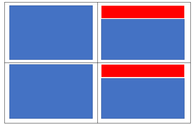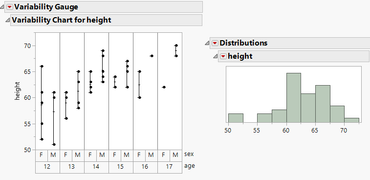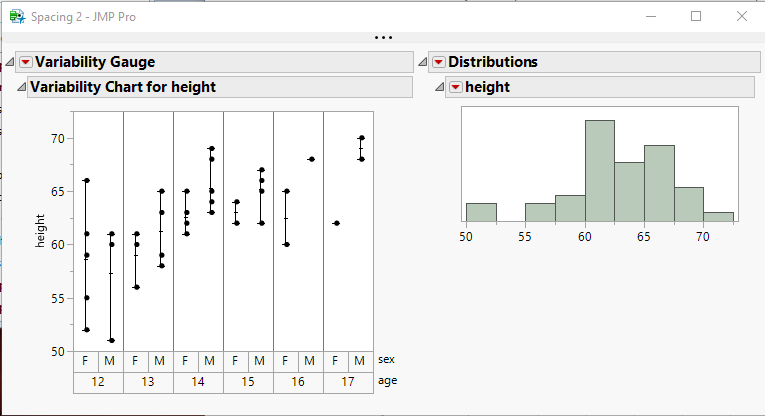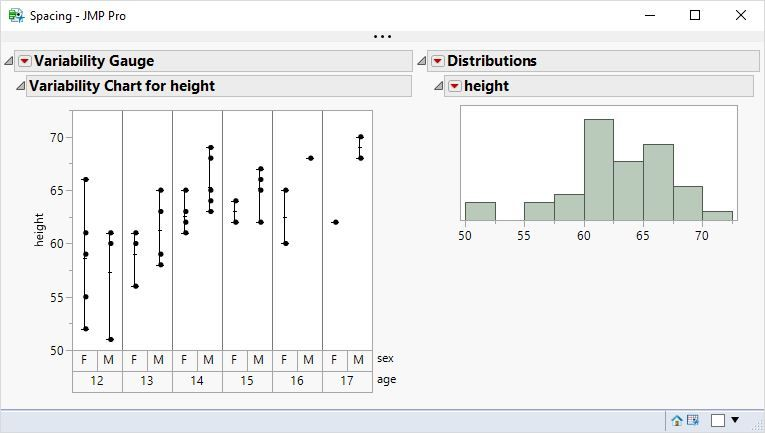Our World Statistics Day conversations have been a great reminder of how much statistics can inform our lives. Do you have an example of how statistics has made a difference in your life? Share your story with the Community!
Choose Language Hide Translation Bar
Highlighted

## Vertical alignment in Lineup Box

Hi,

If I add some display boxes (blue color in the attached image) to a lineup box with two cols,

and the right display box is lower than the left, I get this empty gaps (red color in the attached image).

What is the way to align the children of the lineup to Top?

Thanks

.1 ACCEPTED SOLUTION

Accepted Solutions
Highlighted

## Re: Vertical alignment in Lineup Box

Does this give the behaviour you would like?

``````Names Default To Here( 1 );
Open( "\$SAMPLE_DATA/Big Class.jmp" );

New Window( "Spacing",
Lineup Box( N Col( 2 ), Spacing(0),
v = Variability Chart( Y( :height ), X( :age, :sex ), Std Dev Chart( 0 ) ),
d = Distribution(
Continuous Distribution(
Column( :height ),Quantiles( 0 ),Summary Statistics( 0 ),
Horizontal Layout( 1 ),Vertical( 0 ),Outlier Box Plot( 0 )
)
)
);
);
Wait(2);
Report(v) << verticalAlignment(Top);
Report(d) << verticalAlignment(Top);``````
9 REPLIES 9
Highlighted

## Re: Vertical alignment in Lineup Box

The right side could by another V List Box() that includes the actual display box you want to show followed by a spacer box.

``````Names Default to Here( 1 );

New Window( "Spacing",
Line Up Box( N Col( 2 ),
Outline Box( "one",
Table Box(
String Col Box( "Stuff 1", { "1", "2", "3" } )
)
),
V List Box(
Outline Box( "two",
Table Box(
String Col Box( "Stuff 1", { "1", "2" } )
)
),
Spacer Box()
)
)
);``````
Learn it once, use it forever!
Highlighted

## Re: Vertical alignment in Lineup Box

you may want to try setting the spacing to 0, and then use spacer box() to get the spacing you want. The objects should actually be aligning to the top. What release of JMP are you running?
Jim
Highlighted

## Re: Vertical alignment in Lineup Box

Thank you to you both.

Unfortunatly, doesnt work for me.

Attached image and script.

I am using JMP Pro 15.1``````Names Default To Here( 1 );
Open( "\$SAMPLE_DATA/Big Class.jmp" );

New Window( "Spacing",
Lineup Box( N Col( 2 ), Spacing(0),
Variability Chart( Y( :height ), X( :age, :sex ), Std Dev Chart( 0 ) ),
V List Box(
Distribution(
Continuous Distribution(
Column( :height ),Quantiles( 0 ),Summary Statistics( 0 ),
Horizontal Layout( 1 ),Vertical( 0 ),Outlier Box Plot( 0 )
),
Spacer Box()
)
)
);
);

``````
Highlighted

## Re: Vertical alignment in Lineup Box

Your placement of the Spacer Box and the size of the Spacer Box is all that needed to be changed to get your code to work``````Names Default To Here( 1 );
Open( "\$SAMPLE_DATA/Big Class.jmp" );

New Window( "Spacing",
Lineup Box( N Col( 2 ), Spacing( 0 ),
Variability Chart( Y( :height ), X( :age, :sex ), Std Dev Chart( 0 ) ),
V List Box(
Distribution(
Continuous Distribution(
Column( :height ),
Quantiles( 0 ),
Summary Statistics( 0 ),
Horizontal Layout( 1 ),
Vertical( 0 ),
Outlier Box Plot( 0 )
)
),
Spacer Box( size( 1, 150 ) )
)
)
);``````
Jim
Highlighted

## Re: Vertical alignment in Lineup Box

Thank you Jim.

When I define exact size of the spacer box, and then the user (I'm developing a dashboard) enlarges the Variability chart, the empty space comes back.

I guess there is no "normal" solution...

Highlighted

## Re: Vertical alignment in Lineup Box

Does this give the behaviour you would like?

``````Names Default To Here( 1 );
Open( "\$SAMPLE_DATA/Big Class.jmp" );

New Window( "Spacing",
Lineup Box( N Col( 2 ), Spacing(0),
v = Variability Chart( Y( :height ), X( :age, :sex ), Std Dev Chart( 0 ) ),
d = Distribution(
Continuous Distribution(
Column( :height ),Quantiles( 0 ),Summary Statistics( 0 ),
Horizontal Layout( 1 ),Vertical( 0 ),Outlier Box Plot( 0 )
)
)
);
);
Wait(2);
Report(v) << verticalAlignment(Top);
Report(d) << verticalAlignment(Top);``````
Highlighted

YES! Thanks!
Highlighted

## Re: Vertical alignment in Lineup Box

Why not simply use a horizontal list box?``````Names Default To Here( 1 );
dt = Open( "\$SAMPLE_DATA/Big Class.jmp" );

New Window( "Spacing",
H List Box(
dt << Variability Chart( Y( :height ), X( :age, :sex ), Std Dev Chart( 0 ) ),
dt << Distribution(
Continuous Distribution(
Column( :height ),Quantiles( 0 ),Summary Statistics( 0 ),
Horizontal Layout( 1 ),Vertical( 0 ),Outlier Box Plot( 0 )
)
)
)
);``````
Learn it once, use it forever!
Highlighted

## Re: Vertical alignment in Lineup Box

In the real dashboard there wiil be many charts of several kinds and NCols number of the lineup will not be constant.

``Report(v) << verticalAlignment(Top);``

is perfect

Article Labels

There are no labels assigned to this post.